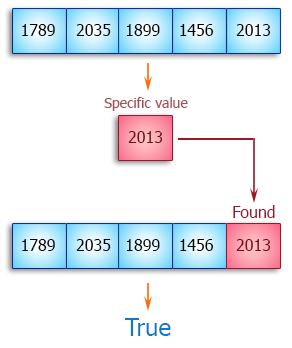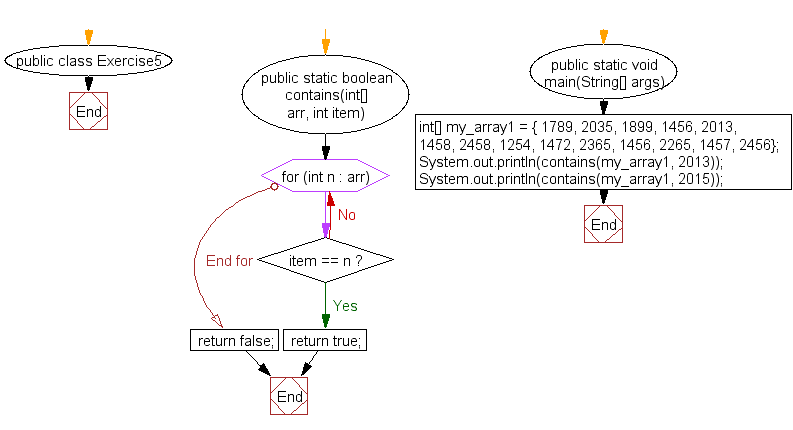﻿ Java exercises: Test if an array contains a specific value - w3resource# Java Array Exercises: Test if an array contains a specific value

## Java Array: Exercise-5 with Solution

Write a Java program to test if an array contains a specific value.

Pictorial Presentation:Sample Solution:

Java Code:

``````public class Exercise5 {
public static boolean contains(int[] arr, int item) {
for (int n : arr) {
if (item == n) {
return true;
}
}
return false;
}
public static void main(String[] args) {
int[] my_array1 = {
1789, 2035, 1899, 1456, 2013,
1458, 2458, 1254, 1472, 2365,
1456, 2265, 1457, 2456};
System.out.println(contains(my_array1, 2013));
System.out.println(contains(my_array1, 2015));
}
}
```
```

Sample Output:

```true
false
```

Flowchart:Visualize Java code execution (Python Tutor):

Java Code Editor:

Improve this sample solution and post your code through Disqus

What is the difficulty level of this exercise?

Test your Programming skills with w3resource's quiz.

﻿

## Java: Tips of the Day

countOccurrences

Counts the occurrences of a value in an array.

Use Arrays.stream().filter().count() to count total number of values that equals the specified value.

```public static long countOccurrences(int[] numbers, int value) {
return Arrays.stream(numbers)
.filter(number -> number == value)
.count();
}
```

Ref: https://bit.ly/3kCAgLb